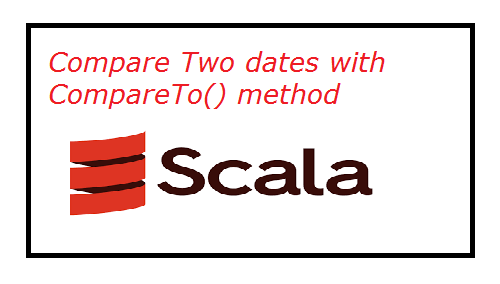Trending Articles       10 Flutter Listview Examples       Flutter Custom Button Example       Flutter Audio Recording       Flutter 2.0 Autocomplete TextField       Current Location Google Maps

# Scala - How to compare two dates

Published June 03, 2021

In this scala example we will cover how to compare two dates programatically. To compare two dates we will use compareTo() method. This compareTo() method return 3 types of values which are

• 0 if the both given dates are equal
• >0 if the current date comes after the given date
• <0 if the current date comes before the given date

Here two compare two dates first we will create two date object by passing the given datesScala example to compare two dates using compareTo() method

 import java.util.Date; object Sample {     def main(args: Array[String]) {          var date1 = new Date(2020, 1, 1);     var date2 = new Date(2021, 6, 1);     var date3 = new Date(2020, 1, 1);     var result: Int = 0;     result = date1.compareTo(date2);     if (result == 0)       println("date1 and date2 are equal");     else if (result > 0)       println("date1 is comes after date2");     else       println("date1 is comes before date2");     result = date1.compareTo(date3);     if (result == 0)       println("date1 and date3 are equal");     else       println("date1 and date3 are not equal");        }   }

The above example will compare given dates and return below output

 ```date1 is comes before date2 date1 and date3 are equal```

 Article Contributed By :76 Views